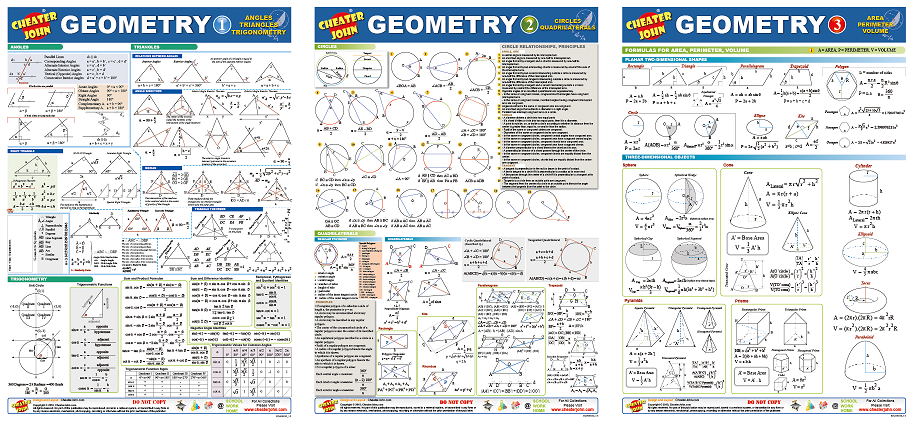# Geometry Formulas and Equations 1-2-3 All in OneAll Geometry Formulas and Equations Cheat Sheet

Geometry formula reference / cheat sheets. For everyday use at school, work and home.

Angles
Type Of Angles

Triangles
Relations Between Angles
Interior Angles, Exterior Angles
Angle Bisectors
Interior Angle Bisector Theorem, Exterior Angle Bisector Theorem
Median
Triangle Theorems
Ceva’s Theorem, Stewart’s Theorem, Basic Proportionality Theorem, Menelaus’ Theorem, Carnot’s Theorem
Right Triangle
Similarity

Trigonometry
Unit Circle
Trigonometric Functions
Sum and Product Formulas
Sum and Difference Identities
Reciprocal, Pythagorean and Quotient Identities
Negative Angle Identities
Trigonometric Function Signs
Trigonometric Values for Common Angles

General
Geometric Symbols

Circles